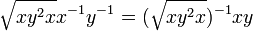# Twisted multiplication of a 2-powered group

## Definition

Suppose$G$ is a 2-powered group, i.e., a group that is powered over the prime number 2, i.e., every element of$G$ has a unique square root. There are two somewhat different but isomorphic definitions of the twisted multiplication.

### The definition that uses square roots on the inside

With the definition:$x *_1 y := \sqrt{x}y\sqrt{x}$

where$\sqrt{u}$ denotes the unique element of$G$ whose square is$u$.

### The definition that uses square roots on the outside

With this definition:$x *_2 y := \sqrt{xy^2x} = \sqrt{(xy)(yx)}$

where$\sqrt{u}$ denotes the unique element of$G$ whose square is$u$.

We can think of this as the "mean" between$xy$ and$yx$. It is the unique solution$z$ to:$z^{-1}xy = z(yx)^{-1}$

### The equivalence of definitions

For any$x,y \in G$, we have:$x^2 *_1 y^2 = (x *_2 y)^2 = xy^2x$

Thus, the square map establishes an isomorphism between the two magma structures.

### Gyrogroup structure

With the twisted multiplication,$G$ acquires the structure of a gyrogroup, in fact, it acquires the structure of a gyrocommutative gyrogroup:

• The identity element is the same as the identity element for$G$ (regardless of whether we are using$*_1$ or$*_2$.
• The inverse operation is the same as the inverse operation of$G$ (regardless of whether we are using$*_1$ or$*_2$.
• In terms of$*_2$, the gyroautomorphism$\operatorname{gyr}([x,y])$ is defined to be conjugation in$G$ by$\sqrt{xy^2x}x^{-1}y^{-1} = (\sqrt{xy^2x})^{-1}xy$. Note that the conjugating element can be thought of as the mean deviation, i.e., it is the distance between either of$xy$ and$yx$ and the mean between them.

## Particular cases

In the case that$G$ is a Baer Lie group (i.e., it is a 2-powered group of nilpotency class two) the twisted multiplication gives rise to an abelian group, and this is the underlying additive group of the Baer Lie ring of$G$ via the Baer correspondence. Another way of formulating this is that the gyroautomorphisms are all identity maps in this case. Note in particular that in this case both the twisted multiplications agree with each other, and both have a nicer alternative description:$x + y := \frac{xy}{\sqrt{[x,y]}}$